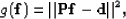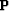Next: Comparing the two methods Up: Multiple subtraction Previous: Pattern-based approach

The goal of adaptive subtraction is to estimate the non-stationary filtersthat minimize the objective function(71)
whererepresents the non-stationary convolution with the multiple model obtained with SRMP (i.e., Chapter) andare the input data. These filters are estimated in a least-squares sense for one shot gather at a time. Note that in practice, a regularization term is usually added in equation () to enforce smoothness between filters. This strategy is similar to the one used in Chapter. The residual vectorcontains the estimated primaries.